Courses

Test: Flood Routing - 2

9 Questions MCQ Test GATE Civil Engineering (CE) 2022 Mock Test Series | Test: Flood Routing - 2

Description
Attempt Test: Flood Routing - 2 | 9 questions in 30 minutes | Mock test for Civil Engineering (CE) preparation | Free important questions MCQ to study GATE Civil Engineering (CE) 2022 Mock Test Series for Civil Engineering (CE) Exam | Download free PDF with solutions
QUESTION: 1

Solution:
QUESTION: 2

In case of channel routing, the storage is a function of

Solution:

Channel routing (Storage is a function of both Inflow & Outflow, here we use Muskingam Method)

QUESTION: 3

In the Muskingum method of channel routing if x = 0.5, it represents an outflow hydrograph

Solution: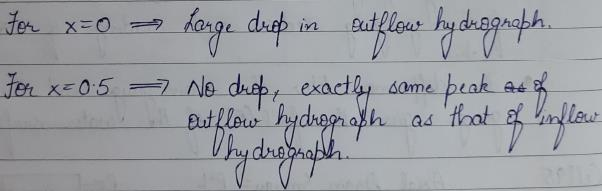QUESTION: 4

Match List-I with List-ll and select the correct answer using the codes given below the lists:
List-I
A. Reservoir routing
B. Hydraulic routing
C. Channel routing
D. Hydrologic routing

List-ll
1. Solution of equation of continuity
2. Solution of St. Venant’s equations
3. Storage is a unique function of outflow discharge
4. Storage is a function of inflow as well as outflow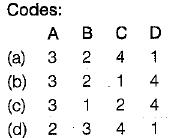Solution:
QUESTION: 5

If the storage S, inflow rate I and outflow rate Q for a river reach is written as
S = K[x In + (1 - x) Qn]

Solution:
QUESTION: 6

If I = inflow rate, Q = outflow rate, and S = storage, in a reach the continuity equation used in flood routing is

Solution:
QUESTION: 7

A linear reservoir is one in which

Solution:
QUESTION: 8

An isochrone is a line on the basin map

Solution:
QUESTION: 9

Which of the following equation is used in hydrological flood routing?

Solution:Use Code STAYHOME200 and get INR 200 additional OFF Use Coupon Code

Track your progress, build streaks, highlight & save important lessons and more!

Similar Content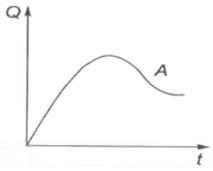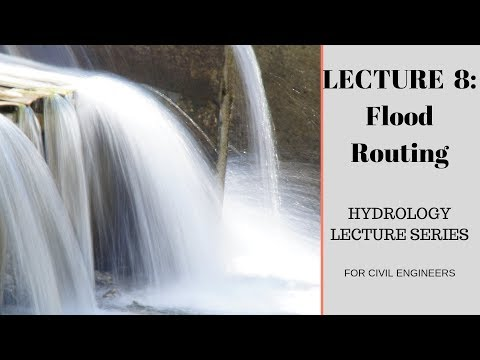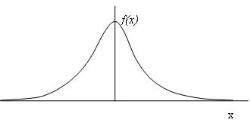Related tests